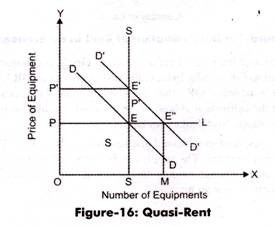# Quasi Rent Theory

The concept of quasi-rent was given by Alfred Marshall. He defined quasi rent as surplus earnings generated by the factors of production, except land.

The earnings from machines and instruments are termed as quasi-rent.

The quasi-rent refers to the income produced when the demand for products increases suddenly.

It is used for a short-period of time. In economic rent, the supply of factor is fixed, such as land. However, in quasi-rent the supply of factor is temporary and can be increased or decreased after some time, such as machine.

For example, there is a sudden increase in the demand of houses, but the supply of houses does not increase with that speed because of the limited building material.

The sudden increase in the return from selling of houses is termed as quasi- rent. Quasi-rent is regarded as the surplus that is temporary in nature. When the building material would be available, then the surplus amount would automatically be eradicated. Similarly, same type of surplus arises in case of other goods, such as ships, machines, and automobiles.

In long-run, the earnings from durable goods are equal to the current interest rate. However, they can provide surplus earnings for temporary period, which are termed as quasi-rent. In short-run, equipment is used for only one purpose and not for other purposes. This implies that the transfer earning for such equipment is zero in the short run.

Therefore, the total earnings generated from the short run equipment are termed as quasi-rent. The supply of equipment is fixed in the short-run and cannot be increased with the increase in demand. However, in long-run, the supply of equipment can be increased that would result in the extinction of surplus earnings.

Quasi rent can also be expressed in terms of revenue, which is as follows:

Quasi-rent = Total revenue – Total variable cost

In the long run, all the costs are considered as variable cost. In long-run, the equilibrium can be attained when total revenue is equal to total costs. In such a case, there is no quasi-rent.

Figure-1 shows the graphical representation of quasi-rent:In Figure 1, SS represents the inelastic supply curve. The demand (DD) and supply (SS) curve intersects at point E. At point E, the price is equal to OP and quantity of equipment is OS. In the short run, the increased demand (D’D’) reaches to the price level of OP’ with the constant supply of OS.

As the number of equipment is constant in short-run, therefore, the transfer earnings are zero and quasi-rent is equal to total earnings from the equipment. However, in long-run, the supply of equipment (PL) is perfectly elastic. Therefore, any number of equipment can be supplied at OP. Now, the supply reaches to OM and prices fall to E”M. The quasi- rent would disappear because the price gets equal to the transfer earning (OP).#### intactone

View all posts by intactone →
error: Content is protected !!